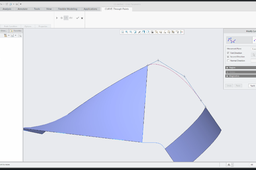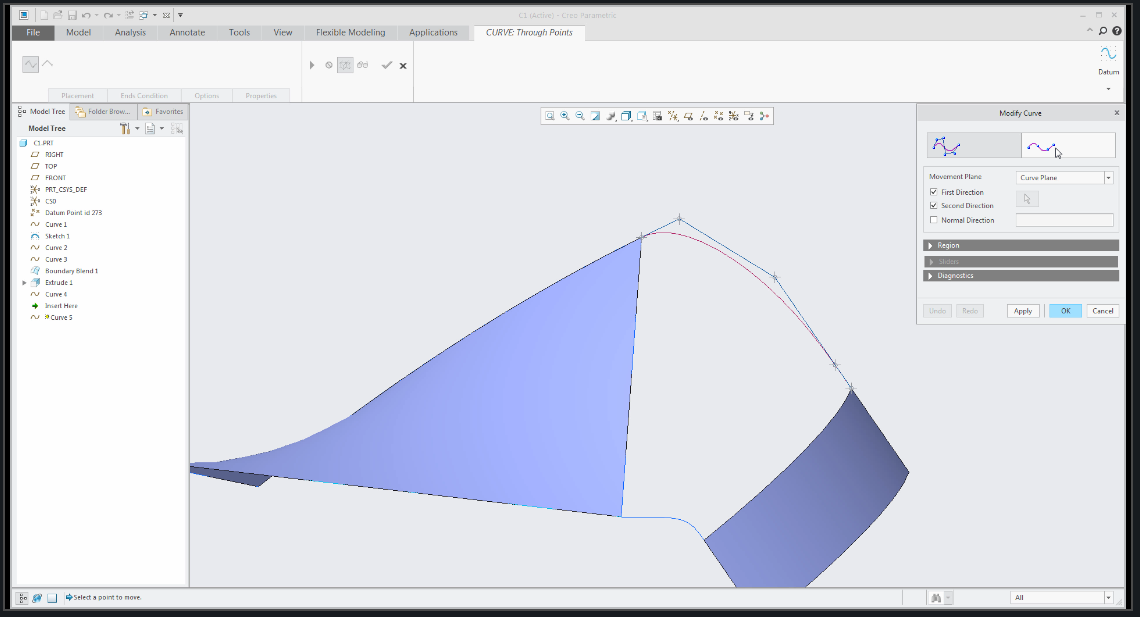# 14 Ways To Create CurveSurface modeling with Creo Parametric 4.0:
14 Ways To Create "Curve"

* Sketched Curve
* 2D Curve created between points
* 3D Curve created by points
* Curve defined by points from CSYS
* Curve conected to another Curves
* Curve created on a surface edge
* Projected Curve
* Wraped Curve
* Curve defined by Equation
* Intersected Curve by curves
* Intersected Curve by quilts
* Copy edge from solid
* Curve from Cross Section
* Trace Curve defined by mechanism

1. ### Step 1: